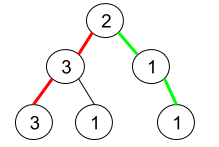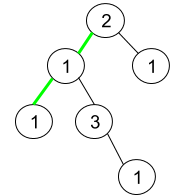Formatted question description: https://leetcode.ca/all/1457.html

# 1457. Pseudo-Palindromic Paths in a Binary Tree (Medium)

Given a binary tree where node values are digits from 1 to 9. A path in the binary tree is said to be pseudo-palindromic if at least one permutation of the node values in the path is a palindrome.

Return the number of pseudo-palindromic paths going from the root node to leaf nodes.

Example 1:Input: root = [2,3,1,3,1,null,1]
Output: 2
Explanation: The figure above represents the given binary tree. There are three paths going from the root node to leaf nodes: the red path [2,3,3], the green path [2,1,1], and the path [2,3,1]. Among these paths only red path and green path are pseudo-palindromic paths since the red path [2,3,3] can be rearranged in [3,2,3] (palindrome) and the green path [2,1,1] can be rearranged in [1,2,1] (palindrome).


Example 2:Input: root = [2,1,1,1,3,null,null,null,null,null,1]
Output: 1
Explanation: The figure above represents the given binary tree. There are three paths going from the root node to leaf nodes: the green path [2,1,1], the path [2,1,3,1], and the path [2,1]. Among these paths only the green path is pseudo-palindromic since [2,1,1] can be rearranged in [1,2,1] (palindrome).


Example 3:

Input: root = 
Output: 1


Constraints:

• The given binary tree will have between 1 and 10^5 nodes.
• Node values are digits from 1 to 9.

Related Topics:
Bit Manipulation, Tree, Depth-first Search

## Solution 1.

A path is pseudo-palindromic if there is at most 1 digit with odd occurrences in the path.

We can use pre-order traversal and keep track of the count of occurrences of digits in cnt array.

If we meet a leaf node and there is at most 1 digit with odd occurrences, we increment the answer.

// OJ: https://leetcode.com/problems/pseudo-palindromic-paths-in-a-binary-tree/

// Time: O(N)
// Space: O(H)
class Solution {
int cnt = {}, ans = 0;
void dfs(TreeNode *root) {
if (!root) return;
cnt[root->val]++;
if (!root->left && !root->right) {
int c = 0;
for (int i = 1; i < 10; ++i) {
c += cnt[i] % 2;
if (c >= 2) break;
}
if (c < 2) ++ans;
}
dfs(root->left);
dfs(root->right);
cnt[root->val]--;
}
public:
int pseudoPalindromicPaths (TreeNode* root) {
dfs(root);
return ans;
}
};


Another variation that doesn’t require the O(9) check of the count of odd occurrences.

// OJ: https://leetcode.com/problems/pseudo-palindromic-paths-in-a-binary-tree/

// Time: O(N)
// Space: O(H)
class Solution {
int cnt = {}, odd = 0, ans = 0;
void dfs(TreeNode *root) {
if (!root) return;
cnt[root->val]++;
int diff = cnt[root->val] % 2 ? 1 : -1;
odd += diff;
ans += !root->left && !root->right && odd < 2;
dfs(root->left);
dfs(root->right);
odd -= diff;
cnt[root->val]--;
}
public:
int pseudoPalindromicPaths (TreeNode* root) {
dfs(root);
return ans;
}
};


Java

/**
* Definition for a binary tree node.
* public class TreeNode {
*     int val;
*     TreeNode left;
*     TreeNode right;
*     TreeNode() {}
*     TreeNode(int val) { this.val = val; }
*     TreeNode(int val, TreeNode left, TreeNode right) {
*         this.val = val;
*         this.left = left;
*         this.right = right;
*     }
* }
*/
class Solution {
public int pseudoPalindromicPaths (TreeNode root) {
if (root == null)
return 0;
if (root.left == null && root.right == null)
return 1;
int count = 0;
Queue<TreeNode> nodeQueue = new LinkedList<TreeNode>();
Queue<String> pathQueue = new LinkedList<String>();
nodeQueue.offer(root);
pathQueue.offer(String.valueOf(root.val));
while (!nodeQueue.isEmpty()) {
TreeNode node = nodeQueue.poll();
String path = pathQueue.poll();
TreeNode left = node.left, right = node.right;
if (left == null && right == null) {
if (canFormPalindrome(path))
count++;
} else {
if (left != null) {
StringBuffer sb = new StringBuffer(path);
sb.append(left.val);
nodeQueue.offer(left);
pathQueue.offer(sb.toString());
}
if (right != null) {
StringBuffer sb = new StringBuffer(path);
sb.append(right.val);
nodeQueue.offer(right);
pathQueue.offer(sb.toString());
}
}
}
return count;
}

public boolean canFormPalindrome(String path) {
Set<Character> set = new HashSet<Character>();
int length = path.length();
for (int i = 0; i < length; i++) {
char c = path.charAt(i);